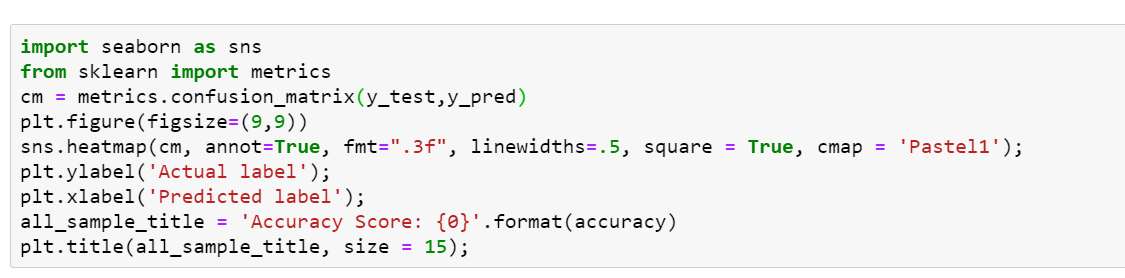# Prerequisites

If you already have Jupyter notebook and all the necessary python libraries and packages installed you are ready to get started.

The Scikit-learn library provides numerous datasets, among which we will be using a data set of images called Digits. This data set consists of 1,797 images that are 8x8 pixels in size. Each image is a handwritten digit in grayscale.

## Analyzing the content

For a textual description of the dataset, the authors who contributed to its creation and the references will appear as shown below.

## Visualizing the images and labels in our Dataset

You can visually check the contents of the results using the matplotlib library.

## Train-test split

Now split the dataset into train and test data. Here my train data size is 75% and the test data size is 25%

# Confusion matrix

A confusion matrix is a table that is often used to evaluate the accuracy of a classification model. We can use Seaborn or Matplotlib to plot the confusion matrix. We will be using Seaborn for our confusion matrix.Using Seaborn to plot out Confusion matrix

# Conclusion

From this article, we can see that the svc estimator has learned correctly and able to recognize the handwritten digits using scikit learn. Also found the accuracy of our prediction(which in our case is 99.33%). I hope this article helps you with your future endeavors!

--

--